# 如何在Python中使用OpenCV进行图像转换？代码示例

2021年11月16日19:15:43 发表评论 669 次浏览

Python如何实现图像转换？本文带你了解如何使用 Python 中的 OpenCV 库执行透视图像转换技术，例如图像平移、反射、旋转、缩放、剪切和裁剪。

## Python OpenCV实现图像转换介绍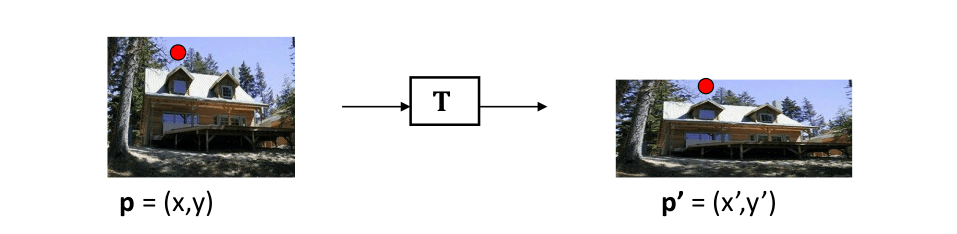例如，如果我们在xy坐标中有(2, 3)个点，而我们在uv坐标中绘制同一个点，同一个点的表示方式不同，如下图所示：

• 图像变换的使用
• 图像平移
• 图像缩放
• 图像剪切
• 在 x 轴方向上剪切
• y 轴方向的剪切
• 图像反射
• 图像旋转
• 图像裁剪
• 结论

## 平移

Python OpenCV实现图像转换：图像平移是图像从一个位置到另一个位置的直线平移，因此物体的平移称为平移。下面显示的矩阵用于图像的平移：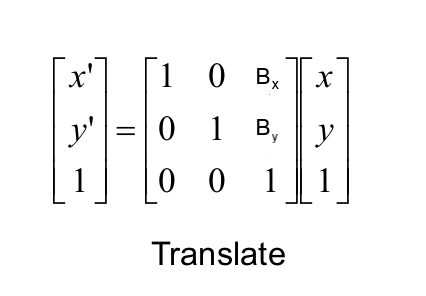x的值 定义了图像在 x 轴上的移动量，而b 的值 决定了图像在 y 轴上的移动：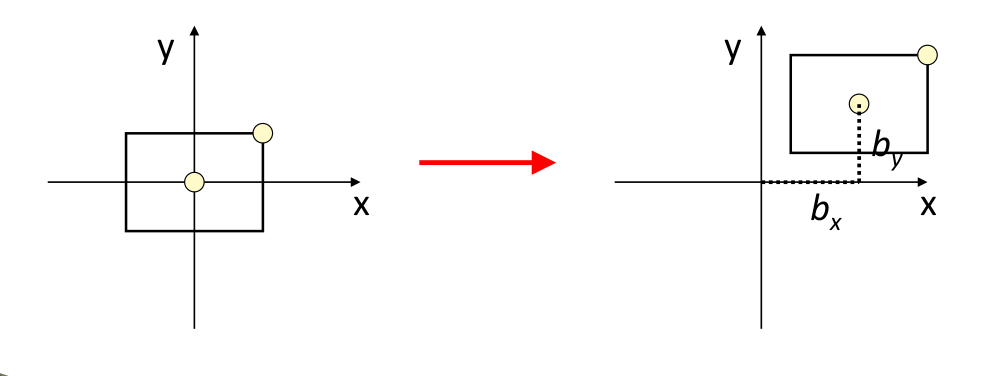现在你了解了图像平移，让我们看一下 Python 代码。在 OpenCV 中，有两个用于执行转换的内置函数：

• `cv2.warpPerspective`: 将(3x3)变换矩阵作为输入。
• `cv2.warpAffine`: 将(2x3)变换矩阵作为输入。

• 输入图像。
• 变换矩阵。
• 图像的高度和宽度的元组。

Python如何实现图像转换？下面的代码读取输入图像（如果你想要确切的输出，请在此处获取演示图像并将其放在当前工作目录中），对其进行平移并显示：

``````import numpy as np
import cv2
import matplotlib.pyplot as plt

# convert from BGR to RGB so we can plot using matplotlib
img = cv2.cvtColor(img, cv2.COLOR_BGR2RGB)
# disable x & y axis
plt.axis('off')
# show the image
plt.imshow(img)
plt.show()
# get the image shape
rows, cols, dim = img.shape
# transformation matrix for translation
M = np.float32([[1, 0, 50],
[0, 1, 50],
[0, 0, 1]])
# apply a perspective transformation to the image
translated_img = cv2.warpPerspective(img, M, (cols, rows))
# disable x & y axis
plt.axis('off')
# show the resulting image
plt.imshow(translated_img)
plt.show()
# save the resulting image to disk
plt.imsave("city_translated.jpg", translated_img)``````

##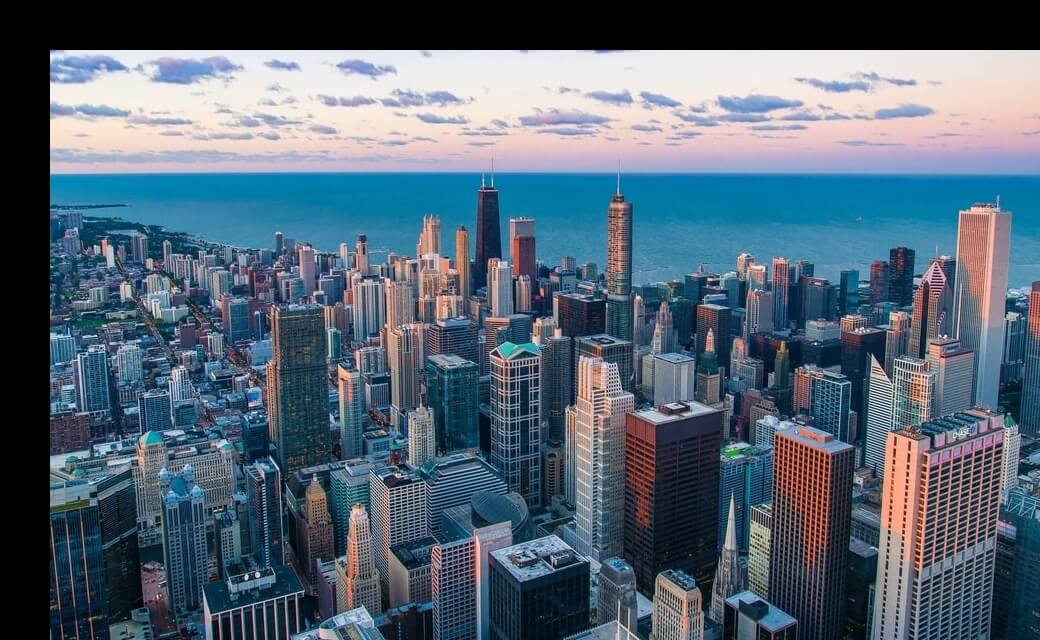图像缩放

Python OpenCV实现图像转换：图像缩放是用于调整数字图像大小的过程。OpenCV 有一个内置函数`cv2.resize()`，但我们将像以前一样使用矩阵乘法来执行转换。用于缩放的矩阵如下所示：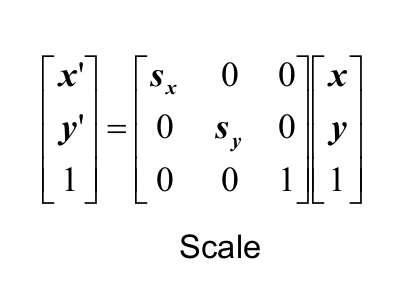x和S y分别是 x 轴和 y 轴的缩放因子。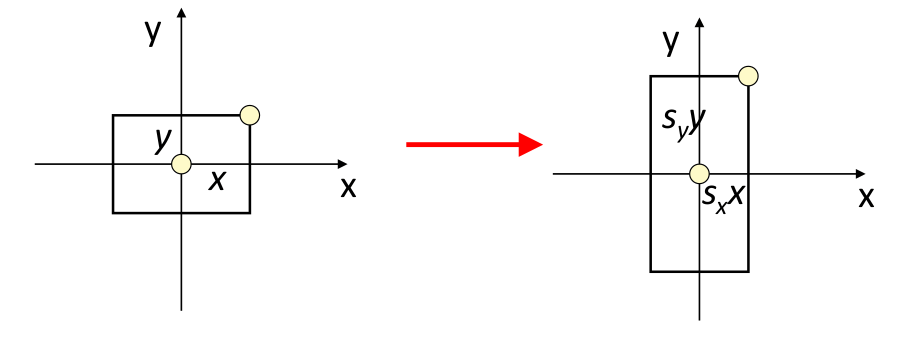下面的代码负责读取相同的图像，定义用于缩放的变换矩阵，并显示结果图像：

``````import numpy as np
import cv2
import matplotlib.pyplot as plt

# convert from BGR to RGB so we can plot using matplotlib
img = cv2.cvtColor(img, cv2.COLOR_BGR2RGB)
# disable x & y axis
plt.axis('off')
# show the image
plt.imshow(img)
plt.show()
# get the image shape
rows, cols, dim = img.shape
#transformation matrix for Scaling
M = np.float32([[1.5, 0  , 0],
[0,   1.8, 0],
[0,   0,   1]])
# apply a perspective transformation to the image
scaled_img = cv2.warpPerspective(img,M,(cols*2,rows*2))
# disable x & y axis
plt.axis('off')
# show the resulting image
plt.imshow(scaled_img)
plt.show()
# save the resulting image to disk
plt.imsave("city_scaled.jpg", scaled_img)``````

## Python图像转换示例：图像剪切

### y 轴方向的剪切

Python如何实现图像转换？在 y 轴方向上进行剪切时，与 y 轴平行的图像边界保持其位置，而与 x 轴平行的边缘根据剪切因子改变其位置。剪切矩阵如下图所示：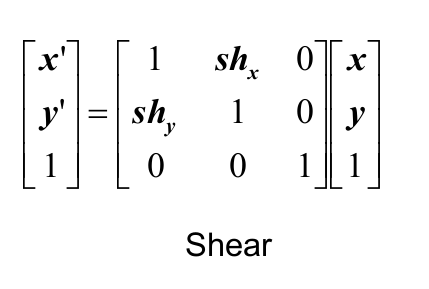下面是负责剪切的代码：

``````import numpy as np
import cv2
import matplotlib.pyplot as plt

# convert from BGR to RGB so we can plot using matplotlib
img = cv2.cvtColor(img, cv2.COLOR_BGR2RGB)
# disable x & y axis
plt.axis('off')
# show the image
plt.imshow(img)
plt.show()
# get the image shape
rows, cols, dim = img.shape
# transformation matrix for Shearing
# shearing applied to x-axis
M = np.float32([[1, 0.5, 0],
[0, 1  , 0],
[0, 0  , 1]])
# shearing applied to y-axis
# M = np.float32([[1,   0, 0],
#             	  [0.5, 1, 0],
#             	  [0,   0, 1]])
# apply a perspective transformation to the image
sheared_img = cv2.warpPerspective(img,M,(int(cols*1.5),int(rows*1.5)))
# disable x & y axis
plt.axis('off')
# show the resulting image
plt.imshow(sheared_img)
plt.show()
# save the resulting image to disk
plt.imsave("city_sheared.jpg", sheared_img)``````

X轴剪切图像：

Y轴剪切图像：

## Python OpenCV实现图像转换：图像反射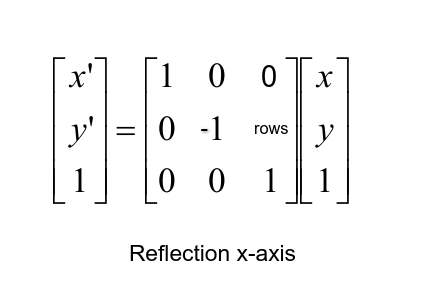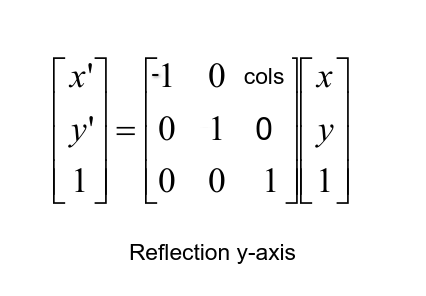这是用于反射的 Python 代码：

``````import numpy as np
import cv2
import matplotlib.pyplot as plt

# convert from BGR to RGB so we can plot using matplotlib
img = cv2.cvtColor(img, cv2.COLOR_BGR2RGB)
# disable x & y axis
plt.axis('off')
# show the image
plt.imshow(img)
plt.show()
# get the image shape
rows, cols, dim = img.shape
# transformation matrix for x-axis reflection
M = np.float32([[1,  0, 0   ],
[0, -1, rows],
[0,  0, 1   ]])
# transformation matrix for y-axis reflection
# M = np.float32([[-1, 0, cols],
#                 [ 0, 1, 0   ],
#                 [ 0, 0, 1   ]])
# apply a perspective transformation to the image
reflected_img = cv2.warpPerspective(img,M,(int(cols),int(rows)))
# disable x & y axis
plt.axis('off')
# show the resulting image
plt.imshow(reflected_img)
plt.show()
# save the resulting image to disk
plt.imsave("city_reflected.jpg", reflected_img)``````

X轴反射图像：

Y轴反射图像：

##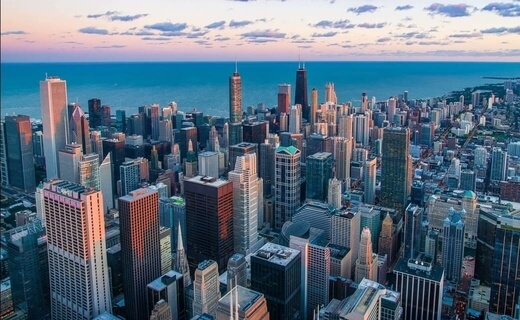(adsbygoogle = window.adsbygoogle || []).push({}); Python图像转换示例：图像旋转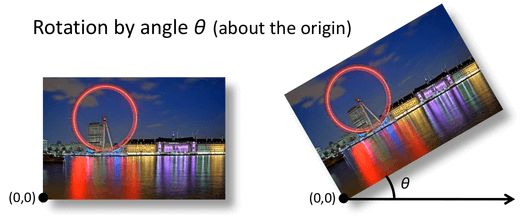旋转变换矩阵如下图所示，其中 theta ( θ ) 为旋转角度：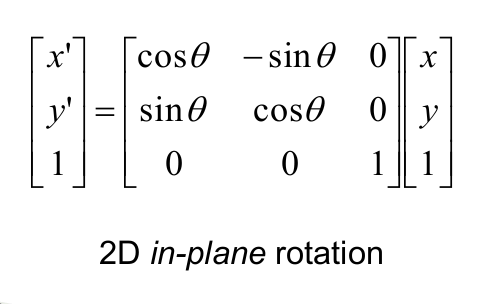下面是图像旋转的 Python 代码：

``````import numpy as np
import cv2
import matplotlib.pyplot as plt

# convert from BGR to RGB so we can plot using matplotlib
img = cv2.cvtColor(img, cv2.COLOR_BGR2RGB)
# disable x & y axis
plt.axis('off')
# show the image
plt.imshow(img)
plt.show()
# get the image shape
rows, cols, dim = img.shape
#transformation matrix for Rotation
M = np.float32([[np.cos(angle), -(np.sin(angle)), 0],
[np.sin(angle), np.cos(angle), 0],
[0, 0, 1]])
# apply a perspective transformation to the image
rotated_img = cv2.warpPerspective(img, M, (int(cols),int(rows)))
# disable x & y axis
plt.axis('off')
# show the resulting image
plt.imshow(rotated_img)
plt.show()
# save the resulting image to disk
plt.imsave("city_rotated.jpg", rotated_img)``````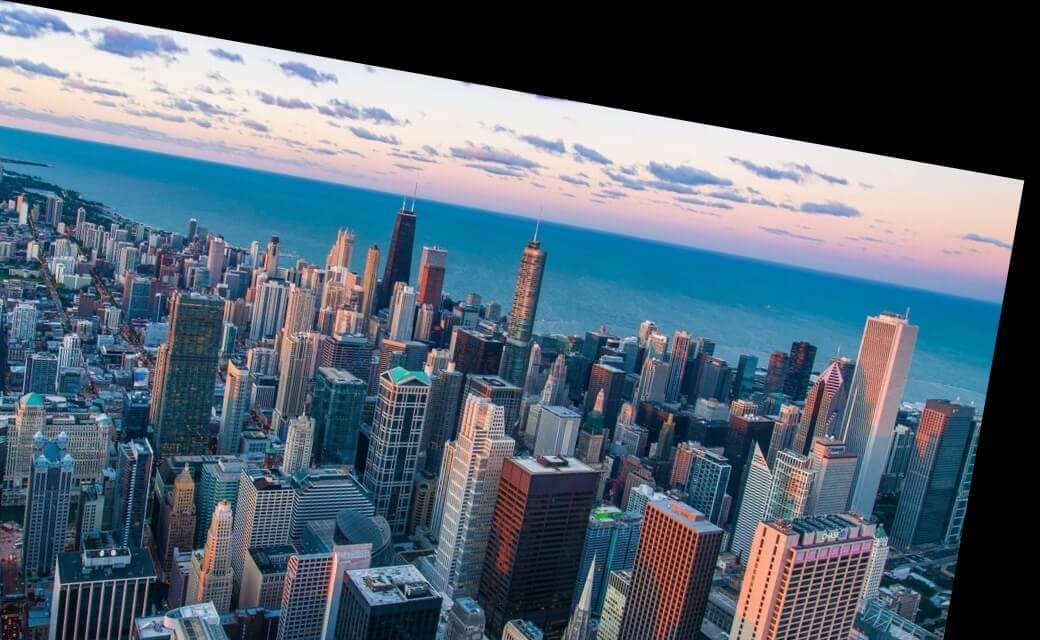这是旋转了 10° ( `np.radians(10)`)，你可以随意编辑它！

## Python图像转换示例：图像裁剪

Python如何实现图像转换？图像裁剪是从图像中去除不需要的外部区域，上面的很多示例都引入了黑色像素，你可以使用裁剪轻松地去除它们。下面的代码是这样做的：

``````import numpy as np
import cv2
import matplotlib.pyplot as plt

# convert from BGR to RGB so we can plot using matplotlib
img = cv2.cvtColor(img, cv2.COLOR_BGR2RGB)
# disable x & y axis
plt.axis('off')
# show the image
plt.imshow(img)
plt.show()
# get 200 pixels from 100 to 300 on both x-axis & y-axis
# change that if you will, just make sure you don't exceed cols & rows
cropped_img = img[100:300, 100:300]
# disable x & y axis
plt.axis('off')
# show the resulting image
plt.imshow(cropped_img)
plt.show()
# save the resulting image to disk
plt.imsave("city_cropped.jpg", cropped_img)``````

Python OpenCV实现图像转换结果解释：由于 OpenCV 将图像加载为 numpy 数组，我们可以简单地通过索引数组来裁剪图像，在我们的例子中，我们选择在两个轴上从100到300获得200 个像素，这是输出图像：

## 结论